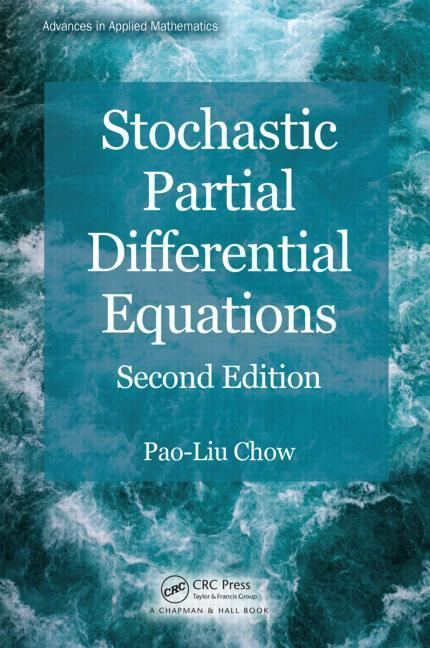# Stochastic partial differential equation

Updated on
Covid-19## Stochastic partial differential equation

Stochastic partial differential equations (SPDEs) generalize partial differential equations via random force terms and coefficients, in the same way ordinary stochastic differential equations generalize ordinary differential equations.

## Contents

They have relevance to quantum field theory and statistical mechanics.

## Examples

One of the most studied SPDEs is the stochastic heat equation, which may formally be written as

t u = Δ u + ξ ,

where Δ is the Laplacian and ξ denotes space-time white noise.

## Discussion

One difficulty is their lack of regularity. In one space dimension, solutions to the stochastic heat equation are only almost 1/2-Hölder continuous in space and 1/4-Hölder continuous in time. For dimensions two and higher, solutions are not even function-valued, but can be made sense of as random distributions.

Stochastic partial differential equation Wikipedia

Topics